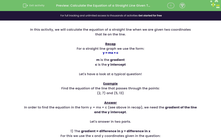# Calculate the Equation of a Straight Line Given Two Coordinates

In this worksheet, students will calculate the equation of a straight line from two given coordinatesKey stage:  KS 3

Curriculum topic:   Algebra

Curriculum subtopic:   Use Coordinates in All Four Quadrants

Difficulty level:#### Worksheet Overview

In this activity, we will calculate the equation of a straight line when we are given two coordinates that lie on the line.

Recap

For a straight line graph we use the form:

## y = mx + c

c is the y intercept

Let's have a look at a typical question!

Example

Find the equation of the line that passes through the points:

(2, 7) and (5, 13)

In order to find the equation in the form y = mx + c (see above in recap), we need the gradient of the line and the y intercept.

1) The gradient = difference in y ÷ difference in x

For this we use the x and y coordinates given in the question:

(2, 7) and (5, 13)

Difference in y ÷ difference in x = {13 - 7} ÷ {5 - 2}

=  6 ÷ 3

=  2

Therefore we know that the equation is y = 2x + c

Let's do part 2 and find the intercept!

2) The y intercept

For this, we can substitute any one of the coordinates into the equation we have so far!

Let's use (2, 7) , so  x = 2 when y = 7

Substitute into the equation:

y = 2x + c

7 =( 2x2) + c

7 = 4 + c

c = 3

The final answer is therefore, y = 2x + 3It seems a bit tricky at first but you will soon pick this up!

Let's try some questions!

### What is EdPlace?

We're your National Curriculum aligned online education content provider helping each child succeed in English, maths and science from year 1 to GCSE. With an EdPlace account you’ll be able to track and measure progress, helping each child achieve their best. We build confidence and attainment by personalising each child’s learning at a level that suits them.

Get started